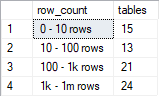## Number of tables by the number of rows in SQL Server database

Article for:

If you want to get an overview on how many rows tables in your database hold one way is to count them by row intervals. This query returns number of tables by the number of their rows grouped into predefined intervals.

## Query

``````select
row_count,
count(*) tables
from
(select
[table],
case when rows > 1000000000 then '1b rows and more'
when rows > 1000000 then '1m - 1b rows'
when rows > 1000 then '1k - 1m rows'
when rows > 100 then '100 - 1k rows'
when rows > 10 then '10 - 100 rows'
else  '0 - 10 rows' end as row_count,
rows as sort
from
(
select schema_name(tab.schema_id) + '.' + tab.name as [table],
sum(part.rows) as [rows]
from sys.tables tab
inner join sys.partitions part
on tab.object_id = part.object_id
where part.index_id IN (1, 0) -- 0 - table without PK, 1 table with PK
group by schema_name(tab.schema_id) + '.' + tab.name
) q
) a
group by row_count
order by max(sort)
``````

## Columns

• row_count - predefined row count intervals:
• 0 - 10 rows
• 10 - 100 rows
• 100 - 1k rows
• 1k - 1m rows
• 1m - 1b rows
• 1b rows and more
• tables - number of tables that row count falls in that interval

## Rows

• One row represents one interval
• Scope of rows: all row count intervals that appear in the database
• Ordered by from smallest tables to the largest

## Sample results

Here is a number of tables by row count in AdventureWorks database aggregated into predefined intervals.